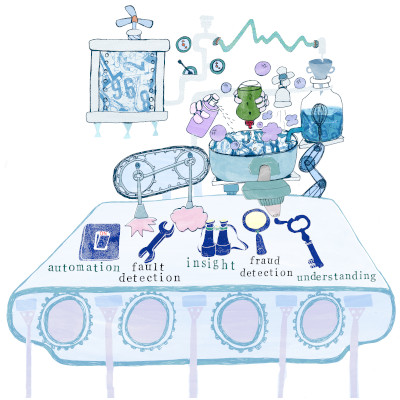GitHub

# PyTorch with PythonCourse Level: Intermediate

Python (along with R) has become the dominant language in machine learning and data science. PyTorch is an open-source machine learning library for Python, based on Torch, used for applications such as natural language processing. It is primarily developed by Facebook’s artificial-intelligence research group, and Uber’s “Pyro” software for probabilistic programming is built on it.

## Book: PyTorch with Python

 Start Date: Price: Venue Details: Time: Duration:

## Course Details

• Course Outline
• Learning Outcomes
• Materials
• Prior Knowledge
• Introduction to machine learning: A brief introduction to the topic of machine learning and the machine learning landscape in Python
• Preprocessing: Building Preprocessing pipelines for data Introduction to deep learning and PyTorch**: An introduction to the PyTorch framework for training deep learning models.
• Building a neural network: Defining a class blueprint for a neural network model in PyTorch
• Training: Understanding feedforward networks, the backpropagation algorithm and optimisation.
• Supervised learning: Building and training models for both regression and classification tasks. Consideration and exploration of different layers and activation functions within a neural network architecture.
• Convolutional neural networks: Learning with image data
• Transfer learning: Leverage the power of existing neural network architectures and weights. This is a powerful technique for training finely tuned models for accomplishing tasks with your own data.
• Deep learning landscape: An exploration of other types of deep learning, the tasks that they aim to solve and how we might implement these in PyTorch. For example unsupervised, reinforcement learning.

On successful completion of the course, delegates will…

• be able to leverage popular python packages such as sklearn and PyTorch for preprocessing their data and creating a pipeline workflow for model building.
• be able to build feed forward neural networks for both regression and classification tasks using the PyTorch package for the Python programming language.
• gain an understanding of deep learning terminology, the mechanisms behind how such networks are trained and an appreciation for the scope of application.
• gain some exposure through discussion to other types of neural networks and time permitting, gain practical experience in other techniques.
•••••The course assumes familiarity with the python programming language including experience of OOP, writing one’s own classes in python. Some knowledge of calculus, matrix algebra and probability would be helpful but not essential.

## Attendee Feedback

• “Very intelligent, approachable trainer combined with clear and concise material”